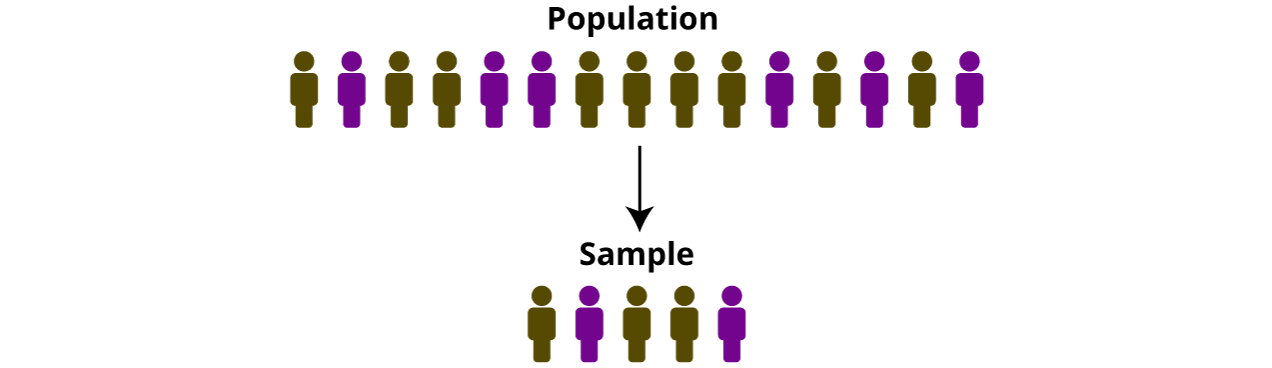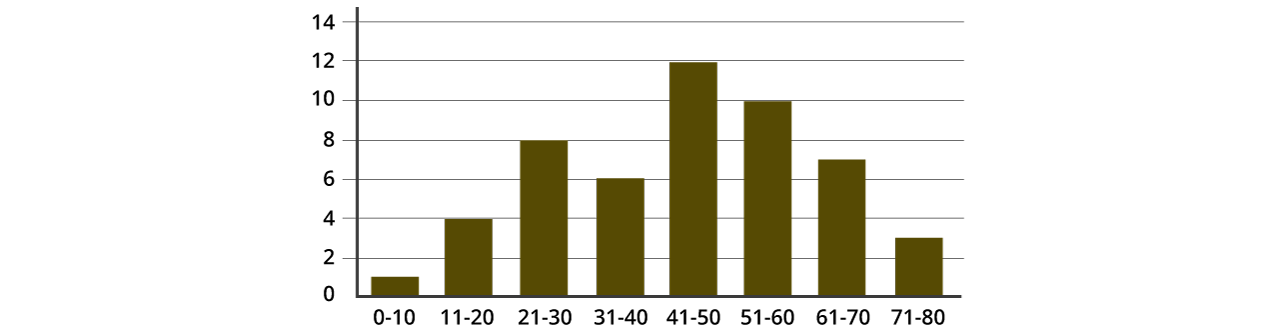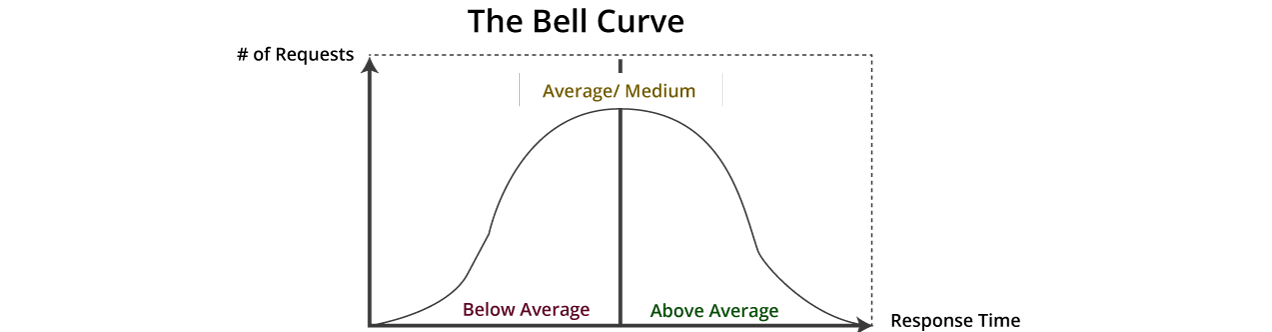Statistics is a science concerned with collection, analysis, interpretation, and presentation of data. In Statistics, we generally want to study a population. You may consider population as a collection of things, persons, or objects under experiment or study. It is usually not possible to gain access to all of the information from the entire population due to logistical reasons. So, when we want to study a population, we generally select a sample.

In sampling, we select a portion (or subset) of the larger population and then study the portion (or the sample) to learn about the population. Data is the result of sampling from a population.## Major Classification

There are two basic branches of Statistics – Descriptive and Inferential statistics. Let us understand the two branches in brief.

### Descriptive statistics

Descriptive statistics involves organizing and summarizing the data for better and easier understanding. Unlike Inferential statistics, Descriptive statistics seeks to describe the data, however, it does not attempt to draw inferences from the sample to the whole population. We simply describe the data in a sample. It is not developed on the basis of probability unlike Inferential statistics.

Descriptive statistics is further broken into two categories – Measure of Central Tendency and Measures of Variability.

### Inferential statistics

Inferential statistics is the method of estimating the population parameter baseon the sample information. It applies dimensions from sample groups in an experiment to contrast the conduct group and make overviews on the large population sample. Please note that the inferential statistics are effective and valuable only when examining each member of the group is difficult.

Let us understand Descriptive and Inferential statistics with the help of an example.

• Task – Suppose, you need to calculate the score othe players who scored a century in a cricket tournament.
•  Solution: Using Descriptive statistics you can get the desired results.
•  Task – Now, you need the overall score of the players who scored a century in the cricket tournament.
• Solution: Applying the knowledge of Inferential statistics will help you in getting your desired results.

## Top Five Considerations for Statistical Data Analysis

Data can be messy. Even a small blunder may cost you a fortune. Therefore, special care when working with statistical data is of utmost importance. Here are a few key takeaways you must consider to minimize errors and improve accuracy.

1. Define the purpose and determine the location where the publication will take place.
2. Understand the assets to undertake the investigation.
3. Understand the individual capability of appropriately managing and understanding the analysis.
4. Determine whether there is a need to repeat the process.
5. Know the expectation of the individuals evaluating reviewing, committee, and supervision.

### Statistics and Parameters

Determining the sample size requires understanding statistics and parameters. The two being very closely related are often confused and sometimes hard to distinguish.

#### Statistics

A statistic is merely a portion of a target sample. It refers to the measure of the values calculated from the population.

A parameter is a fixed and unknown numerical value used for describing the entire population. The most commonly used parameters are:

Mean :

The mean is the average or the most common value in a data sample or a population. It is also referred to as the expected value.

Formula: Sum of the total number of observations/the number of observations.

```Experimental data set: 2, 4, 6, 8, 10, 12, 14, 16, 18, 20
Calculating mean:
(2 + 4 + 6 + 8 + 10 + 12 + 14 + 16 + 18 + 20)/10
= 110/10
= 11 ```

Median:

In statistics, the median is the value separating the higher half from the lower half of a data sample, a population, or a probability distribution. It’s the mid-value obtained by arranging the data in increasing order or descending order.

Formula:

Let n be the data set (increasing order)

When data set is odd: Median = n+1/2th term

```Case-I: (n is odd)
Experimental data set = 1, 2, 3, 4, 5
Median (n = 5) = [(5 +1)/2]th term
= 6/2 term
= 3rd term
Therefore, the median is 3 ```

When data set is even: Median = [n/2th + (n/2 + 1)th] /2

```Case-II: (n is even)
Experimental data set = 1, 2, 3, 4, 5, 6
Median (n = 6) = [n/2th + (n/2 + 1)th]/2
= ( 6/2th + (6/2 +1)th]/2
= (3rd + 4th)/2
= (3 + 4)/2
= 7/2
= 3.5
Therefore, the median is 3.5 ```

Mode:

The mode is the value that appears most often in a set of data or a population.

```Experimental data set= 1, 2, 2, 2, 3, 3, 3, 3, 3, 4, 4,4,5, 6
Mode = 3 ```

(Since 3 is the most repeated element in the sequence.)

## Terms Used to Describe Data

When working with data, you will need to search, inspect, and characterize them. To understand the data in a tech-savvy and straightforward way, we use a few statistical terms to denote them individually or in groups.

The most frequently used terms used to describe data include data point, quantitative variables, indicator, statistic, time-series data, variable, data aggregation, time series, dataset, and database. Let us define each one of them in brief:

• Data points: These are the numerical files formed and organized for interpretations.
• Quantitative variables: These variables present the information in digit form.
• Indicator: An indicator explains the action of a community’s social-economic surroundings.
• Time-series data: The time-series defines the sequential data.
• Data aggregation: A group of data points and data set.
• Database: A group of arranged information for examination and recovery.
• Time-series: A set of measures of a variable documented over a specified time.

## Step-by-Step Statistical Analysis Process

The statistical analysis process involves five steps followed one after another.

• Step 1: Design the study and find the population of the study.
• Step 2: Collect data as samples.
• Step 3: Describe the data in the sample.
• Step 4: Make inferences with the help of samples and calculations
• Step 5: Take action

### Data distribution

Data distribution is an entry that displays entire imaginable readings of data. It shows how frequently a value occurs. Distributed data is always in ascending order, charts, and graphs enabling visibility of measurements and frequencies. The distribution function displaying the density of values of reading is known as the probability density function.

### Percentiles in data distribution

A percentile is the reading in a distribution with a specified percentage of clarifications under it.

Let us understand percentiles with the help of an example.

Suppose you have scored 90th percentile on a math test. A basic interpretation is that merely 4-5% of the scores were higher than your scores. Right? The median is 50th percentile because the assumed 50% of the values are higher than the median.

Dispersion

Dispersion explains the magnitude of distribution readings anticipated for a specific variable and multiple unique statistics like range, variance, and standard deviation. For instance, high values of a data set are widely scattered while small values of data are firmly clustered.

Histogram

The histogram is a pictorial display that arranges a group of data facts into user detailed ranges. A histogram summarizes a data series into a simple interpreted graphic by obtaining many data facts and combining them into reasonable ranges. It contains a variety of results into columns on the x-axis. The y axis displays percentages of data for each column and is applied to picture data distributions.## Bell Curve distribution

Bell curve distribution is a pictorial representation of a probability distribution whose fundamental standard deviation obtained from the mean makes the bell, shaped curving. The peak point on the curve symbolizes the maximum likely occasion in a pattern of data. The other possible outcomes are symmetrically dispersed around the mean, making a descending sloping curve on both sides of the peak. The curve breadth is therefore known as the standard deviation.## Hypothesis testing

Hypothesis testing is a process where experts experiment with a theory of a population parameter. It aims to evaluate the credibility of a hypothesis using sample data. The five steps involved in hypothesis testing are:

• Identify the no outcome hypothesis.

(A worthless or a no-output hypothesis has no outcome, connection, or dissimilarities amongst many factors.)

• Identify the alternative hypothesis.
• Establish the importance level of the hypothesis.
• Estimate the experiment statistic and equivalent P-value. P-value explains the possibility of getting a sample statistic.
• Sketch a conclusion to interpret into a report about the alternate hypothesis.

## Types of variables

A variable is any digit, amount, or feature that is countable or measurable. Simply put, it is a variable characteristic that varies. The six types of variables include the following:

### Dependent variable

A dependent variable has values that vary according to the value of another variable known as the independent variable.

### Independent variable

An independent variable on the other side is controllable by experts. Its reports are recorded and equated.

### Intervening variable

An intervening variable explicates fundamental relations between variables.

### Moderator variable

A moderator variable upsets the power of the connection between dependent and independent variables.

### Control variable

A control variable is anything restricted to a research study. The values are constant throughout the experiment.

### Extraneous variable

Extraneous variable refers to the entire variables that are dependent but can upset experimental outcomes.

## Chi-square test

Chi-square test records the contrast of a model to actual experimental data. Data is unsystematic, underdone, equally limited, obtained from independent variables, and a sufficient sample.

It relates the size of any inconsistencies among the expected outcomes and the actual outcomes, provided with the sample size and the number of variables in the connection.

## Types of Frequencies

Frequency refers to the number of repetitions of reading in an experiment in a given time. Three types of frequency distribution include the following:

• Groupedungrouped
• Cumulative, relative
• Relative cumulative frequency distribution.

## Features of Frequencies

• The calculation of central tendency and position (median, mean, and mode).
• The measure of dispersion (range, variance, and standard deviation).
• Degree of symmetry (skewness).
• Peakedness (kurtosis).

## Correlation Matrix

The correlation matrix is a table that shows the correlation coefficients of unique variables. It is a powerful tool that summarises datasets points and picture sequences in the provided data. A correlation matrix includes rows and columns that display variables. Additionally, the correlation matrix exploits in aggregation with other varieties of statistical analysis.

## Inferential Statistics

Inferential statistics use random data samples for demonstration and to create inferences. They are measured when analysis of each individual of a whole group is not likely to happen.

### Applications of Inferential Statistics

Inferential statistics in educational research is not likely to sample the entire population that has summaries. For instance, the aim of an investigation study may be to obtain whether a new method of learning mathematics develops mathematical accomplishment for all students in a class.

1. Marketing organizations: Marketing organizations use inferential statistics to dispute a survey and request inquiries. It is because carrying out surveys for all the individuals about merchandise is not likely.
2. Finance departments: Financial departments apply inferential statistics for expected financial plan and resources expenses, especially when there are several indefinite aspects. However, economists cannot estimate all that use possibility.
3. Economic planning: In economic planning, there are potent methods like index figures, time series investigation, and estimation. Inferential statistics measures national income and its components. It gathers info about revenue, investment, saving, and spending to establish links among them.

### Key Takeaways

• Statistical analysis is the gathering and explanation of data to expose sequences and tendencies.
•  Two divisions of statistical analysis are statistical and non-statistical analyses.
•  Descriptive and Inferential statistics are the two main categories of statistical analysis. Descriptive statistics describe data, whereas Inferential statistics equate dissimilarities between the sample groups.
•  Statistics aims to teach individuals how to use restricted samples to generate intellectual and precise results for a large group.
•  Mean, median, and mode are the statistical analysis parameters used to measure central tendency.

Conclusion

Statistical analysis is the procedure of gathering and examining data to recognize sequences and trends. It uses random samples of data obtained from a population to demonstrate and create inferences on a group. Inferential statistics applies economic planning with potent methods like index figures, time series investigation, and estimation.  Statistical analysis finds its applications in all the major sectors – marketing, finance, economic, operations, and data mining. Statistical analysis aids marketing organizations in disputing a survey and requesting inquiries concerning their merchandise.